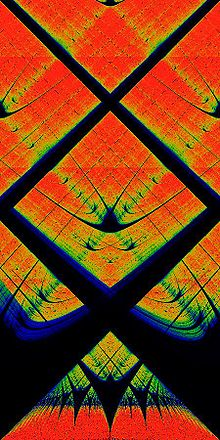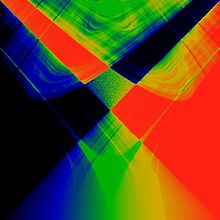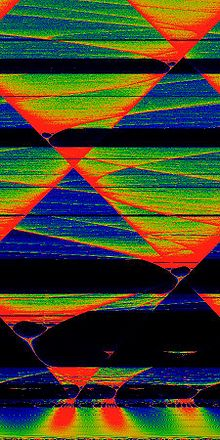# Circle map

﻿
Circle mapCircle map showing mode-locked regions or Arnold tongues in black. Ω varies from 0 to 1 along the x-axis, and K varies from 0 at the bottom to 4π at the top.Rotation number as a function of Ω with K held constant at K = 1Rotation number, with black corresponding to 0, green to 1/2 and red to 1. Ω varies from 0 to 1 along the x-axis, and K varies from 0 at the bottom to 2π at the top.Bifurcation diagram for Ω held fixed at 1/3, and K running from 0 at bottom to 4π at top. Black regions correspond to Arnold tongues.

In mathematics, a circle map is a member of a family of dynamical systems on the circle first defined by Andrey Kolmogorov. Kolmogorov proposed this family as a simplified model for driven mechanical rotors (specifically, a free-spinning wheel weakly coupled by a spring to a motor). The circle map equations also describe a simplified model of the phase-locked loop in electronics. The circle map exhibits certain regions of its parameters where it is locked to the driving frequency (phase-locking or mode-locking in the language of electronic circuits); these are referred to as Arnold tongues, after Vladimir Arnold. Among other applications, the circle map has been used to study the dynamical behaviour of a beating heart.

## Definition

The circle map is given by iterating the map$\theta_{n+1}=\theta_n + \Omega -\frac{K}{2\pi} \sin (2\pi \theta_n).$

where θ is to be interpreted as polar angle such that its value lies between 0 and 1.

It has two parameters, the coupling strength K and the driving phase Ω. As a model for phase-locked loops, Ω may be interpreted as a driving frequency. For K = 0 and Ω irrational, the map reduces to an irrational rotation.

## Mode locking

For small to intermediate values of K (that is, in the range of K = 0 to about K = 1), and certain values of Ω, the map exhibits a phenomenon called mode locking or phase locking. In a phase-locked region, the values θn advance essentially as a rational multiple of n, although they may do so chaotically on the small scale.

The limiting behavior in the mode-locked regions is given by the rotation number$\omega=\lim_{n\to\infty} \frac{\theta_n}{n}.$

which is also sometimes referred to as the map winding number.

The phase-locked regions, or Arnold tongues, are illustrated in black in the figure above. Each such V-shaped region touches down to a rational value Ω = p / q in the limit of$K\to 0$. The values of (K,Ω) in one of these regions will all result in a motion such that the rotation number ω = p / q. For example, all values of (K,Ω) in the large V-shaped region in the bottom-center of the figure correspond to a rotation number of ω = 1 / 2. One reason the term "locking" is used is that the individual values θn can be perturbed by rather large random disturbances (up to the width of the tongue, for a given value of K), without disturbing the limiting rotation number. That is, the sequence stays "locked on" to the signal, despite the addition of significant noise to the series θn. This ability to "lock on" in the presence of noise is central to the utility of phase-locked loop electronic circuit.

There is a mode-locked region for every rational number p / q. It is sometimes said that the circle map maps the rationals, a set of measure zero at K = 0, to a set of non-zero measure for$K\neq 0$. The largest tongues, ordered by size, occur at the Farey fractions. Fixing K and taking a cross-section through this image, so that ω is plotted as a function of Ω gives the Devil's staircase, a shape that is generically similar to the Cantor function.

The circle map also exhibits subharmonic routes to chaos, that is, period doubling of the form 3,6,12,24,....

## Chirikov standard map

The Chirikov standard map is related to the circle map, having similar recurrence relations, which may be written as$\theta_{n+1} = \theta_{n} + p_{n} + {\frac K {2\pi}} \sin(2\pi\theta_{n})$
pn + 1 = θn + 1 − θn

with both iterates taken modulo 1. In essence, the standard map introduces a momentum pn which is allowed to dynamically vary, rather than being forced fixed, as it is in the circle map. The standard map is studied in physics by means of the kicked rotor Hamiltonian.

Wikimedia Foundation. 2010.

### Look at other dictionaries:

• Circle line (London Underground) — Circle Colour on map Yellow Year opened 1884 Line type Sub Surface Rolling stock C Stock 6 carriages per trainset …   Wikipedia

• Circle MRT Line —      Circle MRT Line 地铁环线 The Circle Line is coloured orange on system maps. Overview …   Wikipedia

• Map — /map/, n. Walter, c1140 1209?, Welsh ecclesiastic, poet, and satirist. Also, Mapes /mayps, may peez/. * * * I Graphic representation, drawn to scale and usually on a flat surface, of features usually geographic, geologic, or geopolitical of an… …   Universalium

• Map Collectors' Circle — Formation 1963 Extinction 1975 Type NGO …   Wikipedia

• Circle of fifths — showing major and minor keys …   Wikipedia

• Circle B Bar Reserve — Circle B Bar Reserve …   Wikipedia

• Circle Ranch — U.S. National Register of Historic Places U.S. Historic district …   Wikipedia

• Circle (sculpture) — Circle Artist Sadashi Inuzuka Year 2005 (2005) Type Granite, sand Location …   Wikipedia

• Circle of latitude — Map of Earth Longitude (λ) Lines of longitude appear vertical with varying curvature in this projection, but are actually halves of great ellipses, with identical radii at a given latitude. Latitude (φ) Lines of latitude appear …   Wikipedia